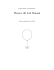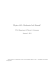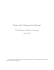# SAMPLE LAB WRITE-UP/PHYSICS 2A & 4A-D Title Objective

## Transcription

SAMPLE LAB WRITE-UP/PHYSICS 2A & 4A-D Title Objective
```SAMPLE LAB WRITE-UP/PHYSICS 2A & 4A-D
Title - Newton’s 2nd Law
Objective
In this experiment we will attempt to confirm the validity of Newton’s 2nd Law by analyzing the
motion of two objects (glider and hanging mass) on a horizontal air-track. First, we will
calculate the theoretical acceleration by applying Newton’s 2nd Law (Fnet = MA), neglecting
friction, to the glider and hanging mass. Next, we will calculate the experimental acceleration of
the glider by applying the kinematic equations of motion as it moves between two markers
(photogates) on the track. We will then compare the experimental acceleration to the theoretical
acceleration.
Theory
a) Acceleration using Newton’s 2nd Law
Apparatus Setup
V1
glider
Photogates
M1
V2
d
+X
Airtrack
M2
+Y
hanging mass
Free-Body Diagram
N
T
M1
T
M1g
M2
M2g
Apply Newton’s 2nd Law to mass M1 and M2.
Mass ‘M1’
ΣFx = T = M1a
Mass ‘M2’
1
ΣFY = M2g - T = M2a
Adding both equations gives:
M2 g = M1 a + M2 a
atheo = M2g/(M1 + M2)
b) Acceleration using Kinematic Equations
Using the kinematic equation V22 = V12 + 2a( x − x0 ) we will calculate the experimental
acceleration of the glider as it moves between the two photogates. We will take the origin of
our coordinate system at the first photogate.
d = distance between photogates
V1 = (s/t1) velocity of the glider through photogate 1
V2 = (s /t2) velocity of the glider through photogate 2
s = diameter of small flag on glider
t1 = time for small flag to go trough photogate 1
t2 = time for small flag to go trough photogate 2
a exp =
V22 − V12
2d
Apparatus
Refer to theory section for apparatus setup
One air track(#21), blower(#2), blower hose and power supply
One digital photogate(#2C) and one accessory photogate(#2A)
One glider(#1B)
One flat accessory box(#22A)
String
Electronic pan balance(#1)
Vernier Calypers (#12c)
Procedure
1.
2.
3.
4.
5.
Measure the mass of the glider and hanging mass.
Setup the air track and blower as indicated by instructor.
Measure the distance between photogates.
Measure the diameter of the small flag on glider with vernier calipers.
Release glider 10 cm away from photogate 1 and record times trough both
photogates.
6. Repeat step (5) four more times.
2
Data
M1= 4750 g
M2=50 g
g = 9.81 m/s2
d = 60.6 cm
s = 1.01 cm
Run #
1
2
3
4
5
t1
0.039
0.043
0.044
0.041
0.038
t2
0.023
0.024
0.023
0.023
0.032
V1 (cm/s)
25.5
23.0
22.5
24.5
26.0
V2 (cm/s)
43.0
41.5
42.5
42.5
43.5
d (cm)
60.6
60.6
60.6
60.6
60.6
a (cm/s2)
9.907
9.862
10.74
9.967
10.05
Calculations
Theoretical Acceleration:
atheo = M2g/(M1 + M2) = 50 g*981 cm/s2/(4750g + 50 g)
atheo = 10.21 cm/s2
Experimental Acceleration:
a exp =
aexp =
V22 − V12 (43.5cm / s ) 2 − (26.0cm / s ) 2
=
= 10.05cm / s 2 (sample calculation Run #5)
2d
2(60.6cm)
9.907 + 9.862 + 1074 + 9.967 + 10.05
= 10.10 cm/s2
5
% error =
% error =
exp− theo
theo
× 100
10.10 − 10.21
10.21
(average experimental acceleration)
× 100 = 1%
3
Conclusion
This experiment confirmed Newton’s 2nd Law. The percent error between experiment and theory
was only 1%. Although the percent error was small there was still some errors involved. These
errors include systematic and random errors:
1. In applying Newton’s 2nd Law to find the acceleration of the glider we neglected the
frictional force. This should have caused the experimental accelerations to be smaller
than the expected theoretical value. The data shows this to be true with the exception of
one data point. Thus, neglecting friction between the glider and the air track introduced a
systematic error in the experiment. We could have eliminated this systematic error by
compensating for friction during the theory derivation.
2. In measuring the velocity of the gliders through the photogates we used the average
velocity instead of the instantaneous velocity. This introduced a systematic error that
may have resulted in the largest source of error in the experiment. If we had a method of
calculating the instantaneous velocity of the glider as it goes through the photogates are
results would have been much better.
3. The random errors involved were due to the uncertainty of the measured quantities.
This includes the uncertainty in the:
a) the length of the small flag
b) the length between the photogates
c) the mass of the glider and hanging mass
To analyze these random errors in detail we need to use the uncertainties in these
measurements and the general error propagation equation to obtain the final acceleration
with its uncertainty. Once we obtain such a numerical result we can check to see if the
expected acceleration falls within the most probable range (MPR) and comment on the
results.
4
```

### Document 6533692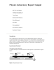### Motion on an Inclined Plane Sample lab report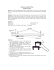### MacGyver E-Collar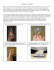### laminate flooring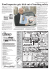### Meadowview Backyard Poly Furniture Catalog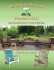### Outdoor Products Brochure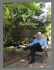### DPF or UREA### Presentation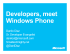### Newport Glider Health Care Series1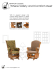### SAFETY GUIDELINES SWING PARTS LIST NEPTUNE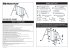### Elastic and Inelastic Collisions: Air Track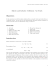### A âgliderâ Observing Task Team (TT) in EuroGOOS: towards the EOOS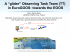### Forces and Newton`s Second Law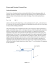### Physics 101 Lab Manual Princeton University m (Revised September 19, 2014)